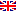Scheda programma d'esame
LABORATORIO SPERIMENTALE DI MATEMATICA COMPUTAZIONALE
DARIO ANDREA BINI
CdSMATEMATICA
Codice062AA
CFU6
PeriodoSecondo semestre
LinguaItaliano

ModuliSettore/iTipoOreDocente/i
LABORATORIO SPERIMENTALE DI MATEMATICA COMPUTAZIONALEMAT/08LABORATORI42
 DARIO ANDREA BINI unimap PAOLA BOITO unimapEsporta in pdf
Learning outcomes
Knowledge

Advanced use of languages like Matlab and Octave. Ability to use these languages to solve mathematical models. Familiarity with the process of creating a mathematical model from problems of the real world.

Assessment criteria of knowledge

Designing  optimized functions in Matlab/Octave and solving different mathematical models and problems with the help of Matlab / Octave

Skills

At the end of the course students will be able to analyze mathematical problems with graphical tools, solving ordinary differential equations modeling  problems in applied sciences, facing problems related to the Web and to image restoration.

Assessment criteria of skills

Designing  optimized functions in Matlab/Octave and completing projects from the level of the modelization of a problem to the design of a solution algorithm and its implementation.

Behaviors

Familiarity with mathematical modeling, with computational aspects, with efficient implementation of the software

Assessment criteria of behaviors

Solving different exercises by analyzing models and designing software

Prerequisites

Basic notions in Linear Algebra, Analysis, Numerical Analysis, basic knowledge of Matlab / Octave

Syllabus

The Page Rank problem and the Page Rank algorithm: the power method. Recommendation systems. The Singular Values Decomposition: application to image compression, application to image recognition. Graphical analysis of Prime numbers: the Ulam spiral and other constructions. Graphical representation of functions of complex variable. Fixed point iterations: creating basins of attraction and fractals, Julia sets. The Arnold cat: a way for image criptography. The Fast Fourier Transform and its application to signal and image filtering. A model for image restoration: point-spread functions and solving large structured systems, the problem of regularization. Numerical solution of ordinary differential equations by means of Matlab, applications to different models from the Real World.

Bibliography

Lecture notes from the lecturers.

Non-attending students info

Students who cannot attend the lectures are requested to solve all the exercises proposed in the class, downloadable from the web page of the course, and an additional project which will be proposed directly by the lecturers.

Assessment methods

Assessment is performed through the correctness of the exercises proposed in the class during the lectures, and through the individual work performed at home.

Pagina web del corso

https://elearning.dm.unipi.it

Ultimo aggiornamento 27/07/2017 15:26